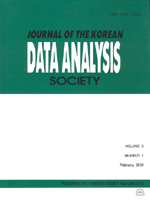상세검색
최근 검색어 전체 삭제
다국어입력
즐겨찾기0KCI등재 학술저널

# A Comparison on the Weighting Scheme in the Censored Linear Regression Model

• 등재여부 : KCI등재
• 2003.12
• 761 - 768 (8 pages)

Kaplan-Meier estimator or its variations are useful for estimating survival distribution and censoring distribution. Particularly, an estimate of censoring distribution function can be used directly to the estimation of parameter of interest with an appropriate weights. In this paper we compare the effects of two weighting schemes proposed by Koul et al. and Zhou, respectively, in the estimation of regression parameter vector for censored linear regression model, where weights are computed by three different types of Kaplan-Meier estimators. Illustration is given by using famous Stanford heart transplant dat set. Stratification of data for the good estimation of regression coefficient was also considered.

1. Introduction

2. Weighting Scheme and Estimates of Censoring distribution

3. Comparison on Two Weighting Schemes

4. Concluding Remarks

References